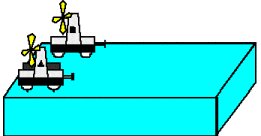# Momentum, Impulse, and KE Question?

I was doing a physics problem on my homework and was not sure which of the three questions below i got wrong as I got a 2/3. I believe the second one might be wrong, but I'm not to sure.

Two fan carts labeled A and B are placed on opposite sides of a table with their fans pointed in the same direction as shown in the figure below. Cart A is weighted with iron bars so it is twice as heavy as cart B. When the fans are turned on, they cause the air to exert a constant force on the cart independent of its mass. Assume that friction is small enough to be neglected. The fans are set with a timer so that after they are switched on, they stay on for a fixed length of time, Δt, and then are turned off.A. Just after the fans are turned off, which of the following statements are true about the momenta of the two carts? pA = pB
B. Just after the fans are turned off, which of the following statements are true about the kinetic energies of the two carts? KEA
C. Which of the following statements are true? The momentum of each cart is conserved.

pA = pB

KEA < KEB

The momentum of each cart is conserved.

Doc Al
Mentor

1. I assumed that constant force and fix time would cause both objects to have equal impulses. With impulse (F*delta T) being equal to momentum, I assumed the two objects would be equal.

2. I did some plug an chug of made up numbers and found that if the mass was twice as large and both objects need to equal the same impulse, then the speed/velocity would be larger for the smaller object.

3. Because of my answer to one I felt that this response is necessary for 1 to be right.

these are the questions and choices
(a) Just after the fans go off, which of the following statements are true about the momenta of the two carts?

• (i) pA > pB
(ii) pA = pB
(iii) pA < pB
(b) Just after the fans go off, which of the following statements are true about the kinetic energies of the two carts?

• (i) KA > KB
(ii) KA = KB
(iii) KA < KB
(c) Which of the following statements are true? You may choose as many as you like, or none. If you choose none, write N.

• (i) After the fan is turned on, each cart moves at a constant velocity,
but the two velocities are different from each other.
(ii) The kinetic energy of each cart is conserved.
(iii) The momentum of each cart is conserved.

Doc Al
Mentor
1. I assumed that constant force and fix time would cause both objects to have equal impulses. With impulse (F*delta T) being equal to momentum, I assumed the two objects would be equal.
Good.

2. I did some plug an chug of made up numbers and found that if the mass was twice as large and both objects need to equal the same impulse, then the speed/velocity would be larger for the smaller object.
Good.

3. Because of my answer to one I felt that this response is necessary for 1 to be right.
Are the initial and final momentums equal?

Good.

Good.

Are the initial and final momentums equal?
I don't think they would be

Doc Al
Mentor
I don't think they would be
Of course not. They start at rest and end up moving with some velocity. So what does that tell you about what is or isn't conserved?

Good.

Good.

just found out there is a forth otpi
Of course not. They start at rest and end up moving with some velocity. So what does that tell you about what is or isn't conserved?
I got it there is a fourth selection, which is that none of them are true.

Doc Al
Mentor
I got it there is a fourth selection, which is that none of them are true.
There you go. Good!

There you go. Good!
Thanks